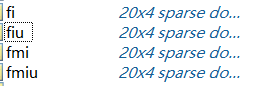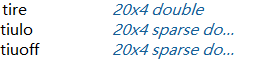# About the case where the result does not satisfy the constraint

I used the CVX solver to show that the problem was solved, but I checked the constraints and they were not met at all. How could this happen

1 Like

Great question. However… it will be appeciative for the benefit of the audience to provide the full results on the type of CVXsolver used such as ‘SeDuMi’ and SDPT3 since you confirm the problem was solved . Do include your codes for better appreciation of your problem

Supra

`````` for i=1:I
sum6(i)=0;
for n=1:N
L6=t0*fi(n,i)/ci(i)+tiulo(n,i)*B*log2(1+Pi(n,i)/(H^2+(norm(u0(:,n)-si(:,i)))^2));
sum6(i)=sum6(i)+L6;
end
sum6(i)==Ii(i);
``````

fi and tiulo are variables, everything else is known, CVX solver tells me I’ve solved it, but both of these variables are zero, whereas all of the elements in Ii are non-zero, obviously that doesn’t satisfy the constraint, why is that?

1 Like
``````    Calling SDPT3 4.0: 2729 variables, 1104 equality constraints
For improved efficiency, SDPT3 is solving the dual problem.
------------------------------------------------------------

num. of constraints = 1104
dim. of sdp    var  = 1280,   num. of sdp  blk  = 640
dim. of linear var  = 792
dim. of free   var  = 17
46 linear variables from unrestricted variable.
*** convert ublk to lblk
number of nearly dependent constraints = 952
To remove these constraints, re-run sqlp.m with OPTIONS.rmdepconstr = 1.
*******************************************************************
SDPT3: Infeasible path-following algorithms
*******************************************************************
version  predcorr  gam  expon  scale_data
HKM      1      0.000   1        0
it pstep dstep pinfeas dinfeas  gap      prim-obj      dual-obj    cputime
-------------------------------------------------------------------
0|0.000|0.000|3.8e+10|2.9e+01|1.4e+12|-3.416443e+09  0.000000e+00| 0:0:00| spchol
SMW too ill-conditioned, switch to LU factor, Inf.
warning: symqmr failed: 0.3
switch to LU factor. splu 30  30
linsysolve: solution contains NaN or inf
stop: difficulty in computing corrector directions
-------------------------------------------------------------------
number of iterations   =  1
primal objective value = -3.41644312e+09
dual   objective value =  0.00000000e+00
gap := trace(XZ)       = 1.39e+12
relative gap           = 4.06e+02
actual relative gap    = -1.00e+00
rel. primal infeas (scaled problem)   = 3.80e+10
rel. dual     "        "       "      = 2.86e+01
rel. primal infeas (unscaled problem) = 0.00e+00
rel. dual     "        "       "      = 0.00e+00
norm(X), norm(y), norm(Z) = 8.6e+02, 0.0e+00, 1.8e+09
norm(A), norm(b), norm(C) = 7.1e+08, 1.0e+00, 6.2e+07
Total CPU time (secs)  = 0.11
CPU time per iteration = 0.11
termination code       =  0
DIMACS: 3.8e+10  0.0e+00  5.9e+01  0.0e+00  -1.0e+00  4.1e+02
-------------------------------------------------------------------

------------------------------------------------------------
Status: Solved
Optimal value (cvx_optval): +0``````
1 Like

Does

Status: Solved
Optimal value (cvx_optval): +0

really go with the preceding solver output? The solver output looks like a disaster, hardly consistent with CVX `Status: Solved`. Perhaps the input data scaling is terrible (some very large magmitude numbers).

Also, when you show only a portion of the program, it makes it more difficult for readers to make reliable conclusion.

1 Like

Hi Dahu . Thanks for the result on exercising SDPT3 solver. However, if you look carefully on it’s response … it’s infeasible as well as ill-conditioned indicating an issue over constraints. As advised by Mark stone it’s best to show your full codes for better appreciation of your problem.

Secondly… can you include your result when you run under ‘SeDuMi’ solver …

Supra

Thank you very much for your opinion, as you said, should I check the constraints or? What is the SeduMi solver you said? Forgive me for using CVX for the first time, I really can’t understand many parts, I will put my code in my reply

1 Like
``````H=50;Vmax=20;z=10;T=5;N=20;t0=T/N;I=4;B=1*10^6;Z=10;c=0.5*Z*t0;
o2=10^-10;om2=10^-10;
y0=10^-2;
c1=100;c2=150;c3=200;c4=250;ci=[c1,c2,c3,c4];
cm1=100;cm2=150;cm3=200;cm4=250;cmi=[cm1,cm2,cm3,cm4];
I1=10000;I2=10000;I3=10000;I4=10000;Ii=[I1,I2,I3,I4];
Im1=10000;Im2=10000;Im3=10000;Im4=10000;Imi=[Im1,Im2,Im3,Im4];
ku=10^-28;k1=10^-28;k2=10^-28;k3=10^-28;k4=10^-28;km1=10^-28;km2=10^-28;km3=10^-28;km4=10^-28;
ki=[k1,k2,k3,k4];ki=repmat(ki,N,1);kmi=[km1,km2,km3,km4];kmi=repmat(kmi,N,1);
p1=0.1;p2=0.1;p3=0.1;p4=0.1;pi=[p1,p2,p3,p4];pi=repmat(pi,N,1);
pm1=10^0.5/100;pm2=10^0.5/100;pm3=10^0.5/100;pm4=10^0.5/100;pmi=[pm1,pm2,pm3,pm4];pmi=repmat(pmi,N,1);
P1=p1*y0/o2;P2=p2*y0/o2;P3=p3*y0/o2;P4=p4*y0/o2;Pi=[P1,P2,P3,P4];Pi=repmat(Pi,N,1);
s1=[5;5];s2=[5;-5];s3=[-5;-5];s4=[-5;5];si=[s1,s2,s3,s4];
sm1=[20;20];sm2=[20;-20];sm3=[-20;-20];sm4=[-20;20];smi=[sm1,sm2,sm3,sm4];
u0=[[-10;0],[-8;0],[-6;0],[-4;0],[-2;0],[0;0],[2;0],[4;0],[6;0],[8;0],[6;0],[4;0],[2;0],[0;0],[-2;0],[-4;0],[-6;0],[-8;0],[-10;0],[-10;0]];
q0=[-10;0];qF=q0;
hm1=(10^-2)*y0*(norm(si(:,1)-smi(:,1)))^-4*pm1/om2;hm2=(10^-2)*y0*(norm(si(:,2)-smi(:,2)))^-4*pm2/om2;hm3=(10^-2)*y0*(norm(si(:,3)-smi(:,3)))^-4*pm3/om2;hm4=(10^-2)*y0*(norm(si(:,4)-smi(:,4)))^-4*pm4 /om2;
hmi=[hm1,hm2,hm3,hm4];hmi=repmat(hmi,N,1);
cvx_begin
variables fi(N,I) fmi(N,I) fiu(N,I) fmiu(N,I);
variables tire(N,I) tiulo(N,I) tiuoff(N,I);
expression sum31(N,I);
expression sum32(N,I);
expression sum41(N,I);
expression sum42(N,I);
expression sum3_i(I);
expression sum3_uav(I);
expression sum4_i(I);
expression sum4_uav(I);
expression sum5(I);
expression sum6(I);
minimize(sum(sum(t0*ki.*pow_p(fi,3)))+sum(sum(tiuoff.*pi))+sum(sum(tiulo.*pi))+sum(sum(t0*kmi.*pow_p(fmi,3)))+sum(sum(tire.*pmi))+sum(sum(t0*ku*pow_p(fiu,3)))+sum(sum(t0*ku*pow_p(fmiu,3))))
subject to:
sum31(1,1)=0;sum31(1,2)=0;sum31(1,3)=0;sum31(1,4)=0;sum32(1,1)=0;sum32(1,2)=0;sum32(1,3)=0;sum32(1,4)=0;
sum41(1,1)=0;sum41(1,2)=0;sum41(1,3)=0;sum41(1,4)=0;sum42(1,1)=0;sum42(1,2)=0;sum42(1,3)=0;sum42(1,4)=0;
%（1）
tire+tiuoff+tiulo==t0/I;
%（2）
for i=1:I
for n=1:N
Gm2=B*log2(1+pmi(n,i)*hmi(n,i)/om2);G2=B*log2(1+Pi(n,i)/(H^2+(norm(u0(:,n)-si(:,i)))^2));
tire(n,i)*Gm2-tiuoff(n,i)*G2<=0;
end
end
%（3）
for i=1:I
for k=2:N
sum31(k,i)=0;sum32(k,i)=0;
for n=2:k
G3=B*log2(1+Pi(n,i)/(H^2+(norm(u0(:,n)-si(:,i)))^2));
sum31(k,i)=sum31(k,i)+tiulo(n,i)*G3;
E3=t0*fiu(n,i)/ci(i);
sum32(k,i)=sum32(k,i)+E3;
end
sum31(k,i)+tiulo(1,i)*B*log2(1+Pi(1,i)/(H^2+(norm(u0(:,1)-si(:,i)))^2))-tiulo(k,i)*B*log2(1+Pi(k,i)/(H^2+(norm(u0(:,k)-si(:,i)))^2))-sum32(k,i)<=0;
end
end
%（4）
for i=1:I
for k=2:N
sum41(k,i)=0;sum42(k,i)=0;
for n=2:k
G4=B*log2(1+Pi(n,i)/(H^2+(norm(u0(:,n)-si(:,i)))^2));
sum41(k,i)=sum41(k,i)+tiuoff(n,i)*G4;
E4=t0*fmiu(n,i)/cmi(i);
sum42(k,i)=sum42(k,i)+E4;
end
sum41(k,i)+tiuoff(1,i)*B*log2(1+Pi(1,i)/(H^2+(norm(u0(:,1)-si(:,i)))^2))-tiuoff(k,i)*B*log2(1+Pi(k,i)/(H^2+(norm(u0(:,k)-si(:,i)))^2))-sum42(k,i)<=0;
end
end
%3,4
for i=1:I
sum3_i(i)=0;sum3_uav(i)=0;
for n=1:N
sum3_i(i)=sum3_i(i)+tiulo(n,i)*B*log2(1+Pi(n,i)/(H^2+(norm(u0(:,n)-si(:,i)))^2));
sum3_uav(i)=sum3_uav(i)+t0*fiu(n,i)/ci(i);
end
sum3_i(i)==sum3_uav(i);
end
for i=1:I
sum4_i(i)=0;sum4_uav(i)=0;
for n=1:N
sum4_i(i)=sum4_i(i)+tiuoff(n,i)*B*log2(1+Pi(n,i)/(H^2+(norm(u0(:,n)-si(:,i)))^2));
sum4_uav(i)=sum4_uav(i)+t0*fmiu(n,i)/cmi(i);
end
sum4_i(i)==sum4_uav(i);
end
%（5）
for i=1:I
sum5(i)=0;
for n=1:N
L5=t0*fmi(n,i)/cmi(i)+tire(n,i)*B*log2(1+pmi(n,i)*hmi(n,i)/om2);
sum5(i)=sum5(i)+L5;
end
sum5(i)==Imi(i);
end
%（6）
for i=1:I
sum6(i)=0;
for n=1:N
L6=t0*fi(n,i)/ci(i)+tiulo(n,i)*B*log2(1+Pi(n,i)/(H^2+(norm(u0(:,n)-si(:,i)))^2));
sum6(i)=sum6(i)+L6;
end
sum6(i)==Ii(i);
end
%（7）
for i=1:I
tire(N,i)==0;tiuoff(N,i)==0;tiulo(N,i)==0;fiu(1,i)==0;fmiu(1,i)==0;
end
%（8）
0<=fi;0<=fmi;0<=fiu;0<=fmiu;0<=tire;0<=tiulo;0<=tiuoff;
cvx_end
``````

The result is as follows
Calling SDPT3 4.0: 2729 variables, 1104 equality constraints
For improved efficiency, SDPT3 is solving the dual problem.
------------------------------------------------------------

`````` num. of constraints = 1104
dim. of sdp    var  = 1280,   num. of sdp  blk  = 640
dim. of linear var  = 792
dim. of free   var  = 17
46 linear variables from unrestricted variable.
*** convert ublk to lblk
number of nearly dependent constraints = 952
To remove these constraints, re-run sqlp.m with OPTIONS.rmdepconstr = 1.
*******************************************************************
SDPT3: Infeasible path-following algorithms
*******************************************************************
version  predcorr  gam  expon  scale_data
HKM      1      0.000   1        0
it pstep dstep pinfeas dinfeas  gap      prim-obj      dual-obj    cputime
-------------------------------------------------------------------
0|0.000|0.000|2.1e+10|2.9e+01|1.4e+12|-3.416443e+09  0.000000e+00| 0:0:00| spchol
SMW too ill-conditioned, switch to LU factor, Inf.
warning: symqmr failed: 0.3
switch to LU factor. splu 30  30
linsysolve: solution contains NaN or inf
stop: difficulty in computing corrector directions
-------------------------------------------------------------------
number of iterations   =  1
primal objective value = -3.41644312e+09
dual   objective value =  0.00000000e+00
gap := trace(XZ)       = 1.39e+12
relative gap           = 4.06e+02
actual relative gap    = -1.00e+00
rel. primal infeas (scaled problem)   = 2.06e+10
rel. dual     "        "       "      = 2.86e+01
rel. primal infeas (unscaled problem) = 0.00e+00
rel. dual     "        "       "      = 0.00e+00
norm(X), norm(y), norm(Z) = 8.6e+02, 0.0e+00, 1.8e+09
norm(A), norm(b), norm(C) = 7.1e+08, 1.8e+00, 6.2e+07
Total CPU time (secs)  = 0.13
CPU time per iteration = 0.13
termination code       =  0
DIMACS: 3.6e+10  0.0e+00  5.9e+01  0.0e+00  -1.0e+00  4.1e+02
-------------------------------------------------------------------

------------------------------------------------------------
Status: Solved
Optimal value (cvx_optval): +0.150208
``````

Obviously wrong solutionAll zero sparse matrix
Constraints 6 are definitely not satisfied, but why doesn’t the solver report an error or?

I ran this with Mosek, which resulted in infeasible.

I ran this with SeDuMi (`cvx_solver sedumi`), which resulted in (optimization) failed.

I ran this with SDPT3, with the same result as you. I do not understand why CVX reported it as solved. It seems like it should have resulted in failed. I have no explanation for the output discrepancy you reported other than there is a bug somewhere and/or everything id screwed up by the horrible input data

The input data looks terrible (very large and small magnitude numbers), although Mosek didn’t issue any warnings, so perhaps some of the most extreme values canceled (netted) out in the actual formulation - I haven’t put in the effort to check on that.

1 Like

Thank you for your valuable comments. I am also checking the input or modifying the output, but I can’t seem to get the answer I want

1 Like